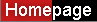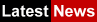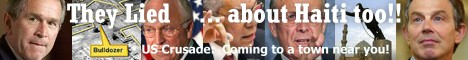9/11 »  PALESTINE »  IRAQ »-- Jump to a Message Board -- RaceandHistory Trinicenter News Rastafari Speaks Trinidad News SupportTHE JET FUEL; HOW HOT DID IT HEAT THE WTC *LINK*

Posted By: September 11 2001
Date: 23, February 03, at 9:34 p.m.

THE JET FUEL; HOW HOT DID IT HEAT THE WORLD TRADE CENTER?

Imagine that the entire quantity of jet fuel from the aircraft was injected into just one floor of the World Trade Center, that the jet fuel burnt with the perfect efficency, that no hot gases left this floor and that no heat escaped this floor by conduction. With these ideal assumptions we calculate the maximum temperature that this one floor could have reached.

"The Boeing 767 is capable of carrying up to 23,980 gallons of fuel and it is estimated that, at the time of impact, each aircraft had approximately 10,000 gallons of unused fuel on board (compiled from Government sources)."

Quote from the FEMA report into the collapse of WTC's One and Two (Chapter Two).

Since the aircraft were only flying from Boston to Los Angeles, they would have been nowhere near fully fueled on takeoff (the aircraft have a maximum range of 7,600 miles). They would have carried just enough fuel for the trip together with some safety factor. Remember, that carrying excess fuel means higher fuel bills and less paying passengers. The aircraft would have also burnt some fuel between Boston and New York.

What we propose to do, is to pretend that the entire 10,000 gallons of jet fuel was injected into just one floor of the World Trade Center, that the jet fuel burnt with the perfect quantity of oxygen, that no hot gases left this floor and that no heat escaped this floor by conduction. With these ideal assumptions (none of which were meet in reality) we will calculate the maximum temperature that this one floor could have reached. Of course, on that day, the real temperature rise of any floor due to the burning jet fuel, would have been considerably lower than the rise that we calculate, but this estimate will enable us to demonstrate that the "official" explanations are lies.

Note that a gallon of jet fuel weighs about 3.1 kilograms, hence 10,000 gallons weighs 10,000 x 3.1 = 31,000 kgs.

Jet fuel is a colorless, combustible, straight run petroleum distillate liquid. Its principal uses are as an ingredient in lamp oils, charcoal starter fluids, jet engine fuels and insecticides.

It is also know as, fuel oil #1, kerosene, range oil, coal oil and aviation fuel.

It is comprised of hydrocarbons with a carbon range of C9 - C17. The hydrocarbons are mainly alkanes CnH2n+2, with n ranging from 9 to 17.

It has a flash point within the range 42° C - 72° C (110° F - 162° F).

And an ignition temperature of 210° C (410° F).

Depending on the supply of oxygen, jet fuel burns by one of three chemical reactions:

(1) CnH2n+2 + (3n+1)/2 O2 => n CO2 + (n + 1) H2O

(2) CnH2n+2 + (2n+1)/2 O2 => n CO + (n + 1) H2O

(3) CnH2n+2 + (n+1)/2 O2 => n C + (n + 1) H2O

Reaction (1) only occurs when jet fuel is well mixed with air before being burnt, as for example, in jet engines.

Reactions (2) and (3) occur when a pool of jet fuel burns. When reaction (3) occurs the carbon formed shows up as soot in the flame. This makes the smoke very dark.

In the aircraft crashes at the World Trade Center the collision would have mixed the fuel with the limited amount of air available within the building, quite well, but the combustion would still have been mainly a combination of reactions (2) and (3) as the quantity of oxygen was quite restricted.

Since we do not know the exact quantities of oxygen available to the fire, we will assume that the combustion was perfectly efficient, that is, the entire quantity of jet fuel burnt via reaction (1), even though we know that this was not so. This generous assumption will give a temperature that we know will be higher than the actual temperature of the fire attributable to the jet fuel.

We need to know that the (net) calorific value of jet fuel when burnt via reaction (1) is 42-44 MJ/kg. The calorific value of a fuel is the amount of energy released when the fuel is burnt. We will use the higher value of 44 MJ/kg as this will lead to a higher maximum temperature than the lower value of 42 (and we wish to continue being outrageously generous in our assumptions).

For a cleaner presentation and simpler calculations we will also assume that our hydrocarbons are of the form CnH2n. The dropping of the 2 hydrogen atoms does not make much difference to the final result and the interested reader can easily recalculate the figures for a slightly more accurate result. So we are now assuming the equation:

(4) CnH2n + 3n/2 O2 => n CO2 + n H2O

However, this model, does not take into account that the reaction is proceeding in air, which is only partly oxygen.

Dry air is 79% nitrogen and 21% oxygen (by volume). Normal air has a moisture content from 0 to 4%. We will include the water vapor and the other minor atmospheric gases with the nitrogen.

So the ratio of the main atmospheric gases, oxygen and nitrogen, is 1 : 3.76. In molar terms:

Air = O2 + 3.76 N2.

Because oxygen comes mixed with nitrogen, we have to include it in the equations. Even though it does not react, it is "along for the ride" and will absorb heat, affecting the overall heat balance. Thus we need to use the equation:

(5) CnH2n + 3n/2(O2 + 3.76 N2) => n CO2 + n H2O + 5.64n N2

From this equation we see that the molar ratio of CnH2n to that of the products is:

 CnH2n : CO2 : H2O : N2 = 1 : n : n : 5.64n moles = 14n : 44n : 18n : 28 x 5.64n kgs = 1 : 3.14286 : 1.28571 : 11.28 kgs = 31,000 : 97,429 : 39,857 : 349,680 kgs

In the conversion of moles to kilograms we have assumed the atomic weights of hydrogen, carbon, nitrogen and oxygen are 1, 12, 14 and 16 respectively.

Now each of the towers contained 96,000 (short) tons of steel. That is an average of 96,000/117 = 820 tons per floor. Lets suppose that the bottom floors contained roughly twice the amount of steel of the upper floors (since the lower floors had to carry more weight). So we estimate that the lower floors contained about 1,100 tons of steel and the upper floors about 550 tons = 550 x 907.2 ? 500,000 kgs. We will assume that the floors hit by the aircraft contained the lower estimate of 500,000 kgs of steel. This generously underestimates the quantity of steel in these floors, and once again leads to a higher estimate of the maximum temperature.

Each story had a floor slab and a ceiling slab. These slabs were 207 feet wide, 207 feet deep and 4 (in parts 5) inches thick and were constructed from lightweight concrete. So each slab contained 207 x 207 x 1/3 = 14,283 cubic feet of concrete. Now a cubic foot of lightweight concrete weighs 50kg, hence each slab weighed 714,150 ? 700,000 kgs. Together, the floor and ceiling slabs weighed some 1,400,000 kgs.

So, now we take all the ingredients and estimate a maximum temperature to which they could have been heated by 10,000 gallons of jet fuel. We will call this maximum temperature T. Since the calorific value of jet fuel is 44 MJ/kg. We know that 10,000 gallons = 31,000 kgs of jet fuel

will release 31,000 x 44,000,000 = 1,364,000,000,000 Joules of energy.

This is the total quantity of energy available to heat the ingredients to the temperature T. But what is the temperature T? To find out, we first have to calculate the amount of energy absorbed by each of the ingredients.

That is, we need to calculate the energy needed to raise:

 39,857 kilograms of water vapor to the temperature T° C, 97,429 kilograms of carbon dioxide to the temperature T° C, 349,680 kilograms of nitrogen to the temperature T° C, 500,000 kilograms of steel to the temperature T° C, 1,400,000 kilograms of concrete to the temperature T° C.

To calculate the energy needed to heat the above quantities, we need their specific heats. The specific heat of a substance is the amount of energy needed to raise one kilogram of the substance by one degree centigrade.

SubstanceSpecific Heat [J/kg*C]
Concrete3,300
Steel450
Nitrogen1,038
Water Vapor1,690
Carbon Dioxide 845

Substituting these values into the above, we obtain:

 39,857 x 1,690 x (T - 25) Joules are needed to heat the water vapor from 25° to T° C, 97,429 x 845 x (T - 25) Joules are needed to heat the carbon dioxide from 25° to T° C, 349,680 x 1,038 x (T - 25) Joules are needed to heat the nitrogen from 25° to T° C, 500,000 x 450 x (T - 25) Joules are needed to heat the steel from 25° to T° C, 1,400,000 x 3,300 x (T - 25) Joules are needed to heat the concrete from 25° to T° C.

The assumption that the specific heats are constant over the temperature range 25° - T° C, is a good approximation if T turns out to be relatively small (as it does). For larger values of T this assumption once again leads to a higher maximum temperature (as the specific heat for these substances increases with temperature). We have assumed the initial temperature of the surroundings to be 25° C. The quantity, (T - 25)° C, is the temperature rise.

So the amount of energy needed to raise one floor to the temperature T° C is

= (39,857 x 1,690 + 97,429 x 845 + 349,680 x 1,038 + 500,000 x 450 + 1,400,000 x 3,300) x (T - 25)
= (67,358,300 + 82,327,500 + 362,968,000 + 225,000,000 + 4,620,000,000) x (T - 25) Joules
= 5,357,650,000 x (T - 25) Joules.

Since the amount of energy available to heat this floor is 1,364,000,000,000 Joules, we have that

5,357,650,000 x (T - 25) = 1,364,000,000,000
5,357,650,000 x T - 133,941,000,000 = 1,364,000,000,000

Therefore T = (1,364,000,000,000 + 133,941,000,000)/5,357,650,000 = 280° C (536° F).

So, if we assume a typical office fire at the WTC, then the jet fuel could have only added 280 - 25 = 255° C (at the very most) to the temperature of the fire.

Summarizing:

We have assumed that the entire quantity of jet fuel from the aircraft was injected into just one floor of the World Trade Center, that the jet fuel burnt with the perfect efficency, that no hot gases left this floor and that no heat escaped this floor by conduction.

We have found that it is impossible the jet fuel, by itself, raised the temperature of this floor beyond 280° C (536° F).

Now this temperature is nowhere near high enough to even begin explaining the World Trade Center Tower collapse.

It is not even close to the first critical temperature of 600° C (1,100° F) where steel loses about half its strength and it is nowhere near the quotes of 1500° C that we constantly read about in our lying media.

"In the mid-1990s British Steel and the Building Research Establishment performed a series of six experiments at Cardington to investigate the behavior of steel frame buildings. These experiments were conducted in a simulated, eight-story building. Secondary steel beams were not protected. Despite the temperature of the steel beams reaching 800-900° C (1,500-1,700° F) in three of the tests (well above the traditionally assumed critical temperature of 600° C (1,100° F), no collapse was observed in any of the six experiments."

Quote from the FEMA report (Appendix A).

So, once again, you have been lied to by the media, are you surprised?

THE JET FUEL; HOW HOT DID IT HEAT THE WTC *LINK*
September 11 2001 -- 23, February 03, at 9:34 p.m.
Re: THE JET FUEL; HOW HOT DID IT HEAT THE WTC
Brian -- 11, September 03, at 3:05 p.m.
Re: THE JET FUEL; HOW HOT DID IT HEAT THE WTC *LINK*
Roderick Allen -- 23, September 03, at 12:25 a.m.
Re: THE JET FUEL; HOW HOT DID IT HEAT THE WTC
andersw -- 22, September 06, at 12:37 p.m.